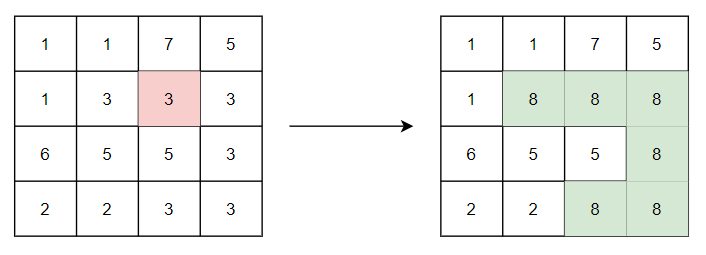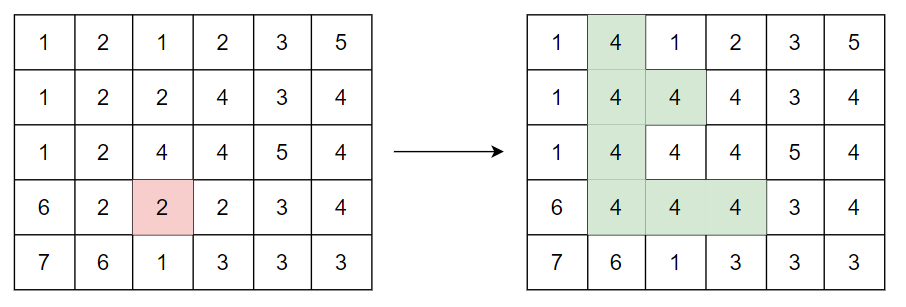7

# Flood Fill Algorithm

Difficulty: MEDIUMContributed By
Anish De
Avg. time to solve
10 min
Success Rate
90%

Problem Statement

#### The image is represented in the form of a 2D array of size M * N. Each pixel in the image is a positive integer. Ninja has given you the coordinates (row and column) of a certain pixel and the new color value. You need to replace the color of the given pixel and all adjacent same-colored pixels with the new color.

##### Note:
``````Two pixels are adjacent if they are connected to each other in any of the four directions: up, down, left, or right.

Diagonal pixels are not considered adjacent.
``````
##### Example:
``````Consider the image of size 4*4, shown below (left). Let the coordinates of the starting pixel are (1, 2) and the new color is 8. The starting pixel, highlighted with red color, has a pixel value of 3.

On replacing the given pixel and all adjacent same-colored pixels with the new color we get the new image, shown below (right). The modified pixels are highlighted with green color.
``````##### Input Format:
``````The first line of input contains an integer ‘T’ representing the number of test cases.

The first line of every test case contains two space-separated integers ‘M’ and ‘N’ representing the number of rows and columns in the image.

Each of the next ‘M’ lines contains ‘N’ space-separated integers denoting the pixel values of the image.

The next line contains three space-separated integers ‘X’, ‘Y’, and ‘C’ denoting the row and column of the starting pixel and the new color, respectively.
``````
##### Output Format:
``````For each test case, the newly colored image is printed in the form of an M * N Matrix.
``````
##### Note:
``````You do not need to print anything, it has already been taken care of. Just implement the given function.
``````
##### Constraints:
``````1 <= T <= 100
1 <= M, N <= 50
0 <= X < M
0 <= Y < N
1 <= Image[i][j], C <= 10^5

Time Limit: 1 sec
``````
##### Sample Input 1:
``````2
4 4
1 1 7 5
1 3 3 3
6 5 5 3
2 2 3 3
1 2 8
5 6
1 2 1 2 3 5
1 2 2 4 3 4
1 2 4 4 5 4
6 2 2 2 3 4
7 6 1 3 3 3
3 2 4
``````
##### Sample Output 1:
``````1 1 7 5
1 8 8 8
6 5 5 8
2 2 8 8
1 4 1 2 3 5
1 4 4 4 3 4
1 4 4 4 5 4
6 4 4 4 3 4
7 6 1 3 3 3
``````
##### Explanation For Sample Input 1:
``````For the first test case, refer to the example explained before.

For the second test case, we are given an image of size 5*6, shown below (left). The coordinates of the starting pixel are (3, 2) and the new color is 4. The starting pixel, highlighted with red color, has a pixel value of 2.

On replacing the given pixel and all adjacent same colored pixels with the new color we get the new image, shown below (right). The modified pixels are highlighted with green color.
``````##### Sample Input 2:
``````2
1 1
5
0 0 6
2 2
1 3
3 3
1 1 5
``````
##### Sample Output 2:
``````6
1 5
5 5
``````Console